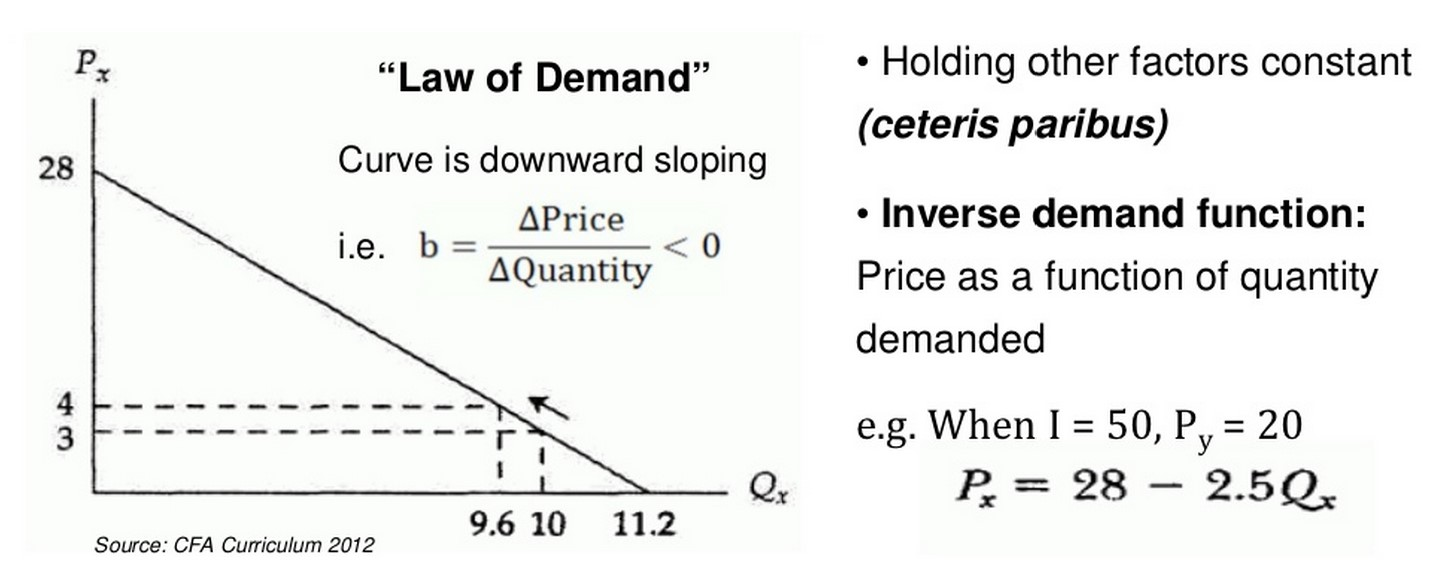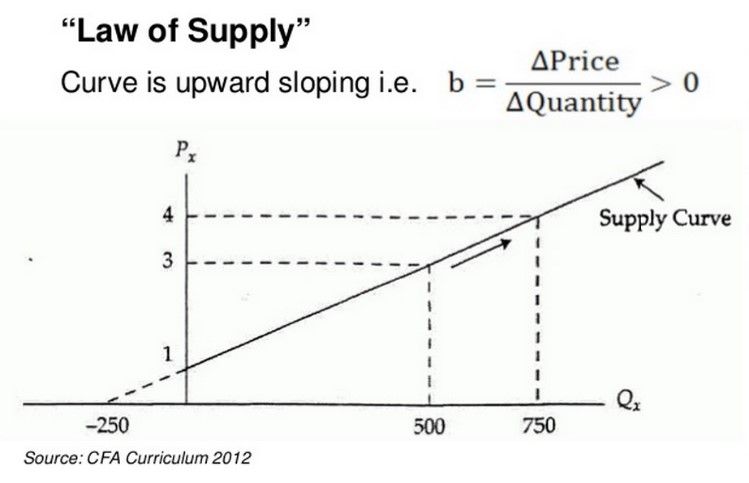## Supply and Demand for the CFA Level 1 Exam

Economics takes up almost 20% of the total curriculum pages within the CFA Level 1 text. And the building block of economics is supply and demand.

To pass the exam you’ll need a clear understanding of supply and demand (the curves) and the concept of equilibrium as well as what causes deviations from equilibrium. Pay particular attention to the calculations of producer and consumer surplus as well as the elasticity calculations as they will all be tested.

## What is the Law of Demand?

Demand is the willingness and ability of consumers to buy a good at a given price.

The law of demand states that, all things being equal, the demand for a good increases (decreases) as the price of that good decreases (increases).

### What Factors does Demand Depend on?

The relationship with related goods is a bit more complicated because it depends on the type of good we are talking about.

Substitute goods, or goods we could use instead of our original item, have a direct relationship with demand. The lower the price of substitute goods the lower demand for my product will be and vice versa. For example if the price of Pepsi drops relative to the price of Coca-Cola, I might drink Pepsi instead of Coke.

Complementary goods are goods which have an inverse relationship with demand of the original product. The lower the price of a complementary good the higher the demand will be. For example, if the price of hamburgers fall people might want to eat more hamburgers which means they’ll buy more hamburger buns.

To summarize:

• The LOWER the price of substitute goods, the LOWER the demand for my product
• The LOWER the price of complementary goods, the HIGHER the demand for my product

We model the general demand function as:

Qdx = f(Px,I,PY)

Where:

Px = The price of good X

I = average individual income

PY = the price(s) of related goods

If we plot that out, with say, the demand for pizza:

Q_d (Pizza)= -120-5p

We can see that if we were to graph the demand function above we would have the price shown as a function of the quantity demanded. That’s a little weird / backwards.

#### Inverting the Demand Function

So we generally invert the demand function to more explicitly show price as a function of the quantity demanded. The Demand curve is the inverse of the demand function with Price on the Y-axis and Quantity Demanded on the X-axis and its slope asThe demand curve shows the highest price consumers are willing to pay for each quantity or alternatively the highest quantity they are willing to purchase at each price.

Movements along the demand curve reflect a change in quantity demanded and are caused by a change in price of the good itself. Shifts of the entire demand curve, either up or down, reflect changes in demand caused by external (non-price) factors such as a change in income, price of complements, price of substitutes, changing tastes or expectations.

For the CFA Level 1 exam you should be comfortable interpreting movements along the demand curve versus wholesale changes in quantity demanded.

## The Law of Supply

Supply measures the willingness and ability of firms to sell a good or service at a given price.

Formally the law of supply states that, all else equal, the quantity of a good supplied varies directly with the price of that good and the cost of producing it. The higher the price, the more of a good suppliers want to sell. The lower the cost of producing a good the more attractive it is to produce it. Both of these should be obvious—the higher the price and the lower the cost to make the greater the ability to make more money!

We model the quantity supplied as being dependent on the selling price, labor costs (wages), and the cost of inputs (raw materials). We model this as

QSx = f(Px,PR,L,K,H)

Where:

QSx = the quantity supplied of good X

Px = the price of good X

PR = the price of related goods

L,K,H = the price of Labor, Capital, and other inputs respectively

#### Inverting the Supply Function

Just like with demand we also invert the supply function in order to generate our upward sloping supply curve. The supply curve shows the relationship between the price and quantity supplied where the slope of the supply curve is equal to:Supply shows the highest price suppliers accept for a given quantity or the highest quantity willingly supplied at a given price.

Movements along the supply curve reflect changes in the quantity supplied as a result of changes in price. Shifts in the supply curve reflect (non-price) related changes such as changes in input costs or technology.

Note that variables specifically incorporated in the supply and demand models are endogenous (price, quantity demanded/suppled). Variables that can impact the model but are not included in it are called exogenous variables.

Summary

It can be helpful to think of shifts of both the supply and demand curves as changes to the independent variables of their respective functions whereas movements along the curves reflect changes to the dependent variable. On the exam be able to take certain facts and determine whether there is (1) a movement along the curve, (2) a shift of a curve, and (3) in which direction the movement or shift occurs.